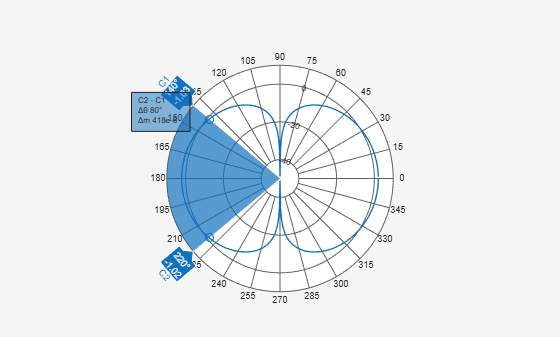Documentation

### This is machine translation

Mouseover text to see original. Click the button below to return to the English version of the page.

Note: This page has been translated by MathWorks. Click here to see
To view all translated materials including this page, select Country from the country navigator on the bottom of this page.

# beamwidth

Beamwidth of antenna

## Syntax

``beamwidth(antenna,frequency,azimuth,elevation)``
``bw = beamwidth(antenna,frequency,azimuth,elevation,dBdown)``
``````[bw,angles] = beamwidth(____)``````

## Description

example

````beamwidth(antenna,frequency,azimuth,elevation)` plots the beamwidth of the input antenna at a specified frequency. The beamwidth is the angular separation at which the magnitude of the directivity pattern decreases by a certain value from the peak of the main beam. The directivity decreases in the direction specified by azimuth and elevation angles of the antenna.```
````bw = beamwidth(antenna,frequency,azimuth,elevation,dBdown)` returns the beamwidth of the antenna at a specified `dBdown` value from the peak of the radiation pattern main beam.```

example

``````[bw,angles] = beamwidth(____)``` returns the beamwidth and angles (points in a plane) using any input arguments from previous syntaxes.```

## Examples

collapse all

Plot the beamwidth for a dipole antenna at azimuth=0 and elevation=1:1:360 (x-z plane)

```d = dipole; beamwidth(d,70e6,0,1:1:360);```Calculate the beamwidth of a helix antenna and the angles of the beamwidth. The antenna has an azimuth angle of 1:1:360 degrees, an elevation angle of 0 degrees on the X-Y plane, and a dB down value of 5 dB.

```hx = helix; [bw,angles] = beamwidth(hx,2e9,1:1:360,0,5)```
```bw = 145 ```
```angles = 1×2 143 288 ```

## Input Arguments

collapse all

Antenna object, specified as a scalar handle.

Frequency to calculate beamwidth, specified as a scalar in Hz.

Example: 70e6

Data Types: `double`

Azimuth angle of the antenna, specified as a scalar or vector in degrees. If the elevation angle is specified as a vector, then the azimuth angle must be a scalar.

Example: 3

Data Types: `double`

Elevation angle of the antenna, specified as a scalar or vector in degrees. If the azimuth angle is specified as a vector, then the elevation angle must be a scalar.

Example: 1:1:360

Data Types: `double`

Power point from peak of main beam of antenna, specified as a scalar in dB.

Example: 5

Data Types: `double`

## Output Arguments

collapse all

Beamwidth of antenna, returned as a scalar in degrees.

Points on plane used to measure beamwidth, returned as a vector in degrees.

## See Also

#### Hybrid Beamforming for Massive MIMO Phased Array Systems

Download the white paper